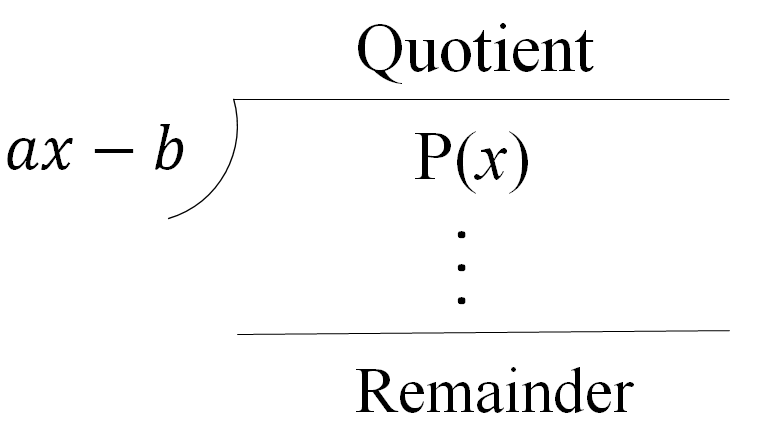# Remainder theorem#### Everything You Need in One Place

Homework problems? Exam preparation? Trying to grasp a concept or just brushing up the basics? Our extensive help & practice library have got you covered.#### Learn and Practice With Ease

Our proven video lessons ease you through problems quickly, and you get tonnes of friendly practice on questions that trip students up on tests and finals.#### Instant and Unlimited Help

Our personalized learning platform enables you to instantly find the exact walkthrough to your specific type of question. Activate unlimited help now!##### Examples
###### Lessons
1. Understanding the remainder Theorem
Prove the Remainder Theorem1. Finding the Remainder Using Synthetic Division and the Remainder Theorem
Find the remainder when $\left( {4{x^3} - 7x + 10} \right)$ is divided by $\left( {2x - 5} \right)$
1. Using synthetic division
2. Using the remainder theorem
2. When $\left( {8{x^3} + a{x^2} + bx - 1} \right)$ is divided by:
i) $\left( {2x - 5} \right)$, the remainder is $54$
ii) $\left( {x + 1} \right)$, the remainder is $- 30$
Find the values of $a$ and $b$.
###### Topic Notes
You may want to refresh your memory on polynomial long division and synthetic division to better understand this lesson. The remainder theorem simply states that if a polynomial f(x) is divided by a linear expression x-r, the value of f(r) is equal to the remainder.
$\cdot$ When a polynomial, $P(x)$, is divided by $(x-a)$: Remainder $=P(a)$
$\cdot$ When a polynomial, $P(x)$, is divided by $(ax-b)$: Remainder $=P(\frac{b}{a})$